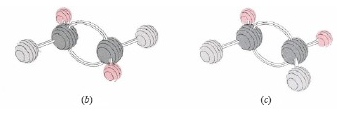# 13.2: The Equilibrium State

$$\newcommand{\vecs}{\overset { \rightharpoonup} {\mathbf{#1}} }$$ $$\newcommand{\vecd}{\overset{-\!-\!\rightharpoonup}{\vphantom{a}\smash {#1}}}$$$$\newcommand{\id}{\mathrm{id}}$$ $$\newcommand{\Span}{\mathrm{span}}$$ $$\newcommand{\kernel}{\mathrm{null}\,}$$ $$\newcommand{\range}{\mathrm{range}\,}$$ $$\newcommand{\RealPart}{\mathrm{Re}}$$ $$\newcommand{\ImaginaryPart}{\mathrm{Im}}$$ $$\newcommand{\Argument}{\mathrm{Arg}}$$ $$\newcommand{\norm}{\| #1 \|}$$ $$\newcommand{\inner}{\langle #1, #2 \rangle}$$ $$\newcommand{\Span}{\mathrm{span}}$$ $$\newcommand{\id}{\mathrm{id}}$$ $$\newcommand{\Span}{\mathrm{span}}$$ $$\newcommand{\kernel}{\mathrm{null}\,}$$ $$\newcommand{\range}{\mathrm{range}\,}$$ $$\newcommand{\RealPart}{\mathrm{Re}}$$ $$\newcommand{\ImaginaryPart}{\mathrm{Im}}$$ $$\newcommand{\Argument}{\mathrm{Arg}}$$ $$\newcommand{\norm}{\| #1 \|}$$ $$\newcommand{\inner}{\langle #1, #2 \rangle}$$ $$\newcommand{\Span}{\mathrm{span}}$$$$\newcommand{\AA}{\unicode[.8,0]{x212B}}$$

A simple and instructive example of a chemical equilibrium is provided by the interconversion of the cis and trans isomers of difluoroethene:

${cis-}\text{C}_{2}\text{H}_{2}\text{F}{2}\rightleftharpoons {trans-}\text{C}_{2}\text{H}_{2}\text{F}_{2} \nonumber$

The two molecules involved in this equilibrium were illustrated in Figure $$\PageIndex{1}$$. The only difference between them is that in the cis isomer the two fluorine atoms are on the same side of the molecule, while in the trans isomer they are on opposite sides of the molecule. Although their molecules are so similar, these two isomers of difluoroethene are distinct chemical substances. They both condense to liquids at low temperatures, but these liquids have different boiling points. At room temperature both are gases, but they may be separated from each other and analyzed quantitatively by the technique of gas chromatography.Figure $$\PageIndex{1}$$ Cis and Trans isomers of difluoroethane.

Other sections discuss that the barrier to free rotation about thebond prevents cis-C2H2F2 from changing rapidly into trans-C2H2F2. The same applies to the reverse reaction, conversion of the trans isomer to the cis. These reactions occur very slowly at higher temperatures, and even at 700 K (427°C), several weeks are required before equilibrium is reached and the concentrations of cis and trans species no longer vary with time. To study the reaction conveniently, a catalyst, such as I2(g) is added, speeding the reaction so that equilibrium is reached in a few minutes. When this is done, we always end up with a mixture which is slightly richer in the cis isomer. Furthermore, at a given temperature, the ratio of concentrations of the two isomers is always the same. For example, at 623 K the ratio

$\frac{\text{Equilibrium concentration of trans}}{\text{Equilibrium concentration of cis}}=\frac{[trans\text{-C}_{2}\text{H}_{2}\text{F}_{2}]}{[cis\text{-C}_{2}\text{H}_{2}\text{F}_{\text{2}}]}=\text{0.50 }\label{2}$

[In the second ratio in Eq. $$\ref{2}$$ square brackets are used to indicate the concentrations of trans C2H2F2 and cis-C2H2F2 once equilibrium has been reached.]Figure $$\PageIndex{2}$$ Chemical equilibrium. When any mixture of cis-and-trans- difluoroethene is heated to 623 K, it eventually changes into an equilibrium mixture of the isomers with 33.3% of the trans isomer and 66.7% of the cis isomer. That is, the ratio [trans-C2H2F2]/[cis-C2H2F2] is always 0.5 at 623 K.

Apart from a change in temperature, nothing will alter this equilibrium ratio from 0.50. Whether we start with the pure cis isomer, the pure trans isomer, or even a mixture of isomers, the same ratio is obtained (see Figure $$\PageIndex{2}$$ ). Other variations, such as starting with half the amount of either isomer, changing the volume of the container, or heating the mixture to 1000 K and then cooling it to 623 K, are likewise without effect. Even adding a catalyst has no effect on the equilibrium ratio. If we heat cis-C2H2F2 and trans-C2H2F2 to 623 K with iodine added as a catalyst, the only difference is that equilibrium is achieved in a few minutes instead of a few weeks. The final composition is the same as in the uncatalyzed case.

We have described this equilibrium between the cis and trans isomers of difluoroethene because it demonstrates very clearly the four features which are characteristic of any chemical situation in which appreciable concentrations of reactants and products are in equilibrium with each other. These four features are

1. Even though the attainment of an equilibrium may be slow, once equilibrium has been achieved, the concentrations of all species participating in the equilibrium remain constant.
2. A chemical equilibrium can always be attained by approaching the equilibrium from more than one direction. We can begin with pure products or with pure reactants. Alternatively we can approach the equilibrium from either a higher or lower temperature.
3. The final equilibrium concentrations of the reactants are unaffected by the presence or absence of a catalyst.
4. There is always some mathematical relationship connecting the concentrations of the various species involved in the equilibrium mixture at a constant temperature. In the example just discussed, the concentrations were in a constant ratio. Usually the relationship is more complex, as we will see in the next section.

It is not always easy to tell when a chemical system is in a genuine equilibrium state. We often find mixtures of substances whose compositions do not change with time but which are not really in equilibrium with each other. A mixture of hydrogen and oxygen gas at room temperature is a good example. Although hydrogen and oxygen do react with each other to form water at room temperature, this reaction is so slow that no detectable change is apparent even after a few years. However, if an appropriate catalyst is added, the two gases react explosively and are converted completely to water according to the equation

$\text{2H}_{2}\text{ }({g}) + \text{O}_{2}\text{ } ({g})\rightarrow \text{2H}_{2}\text{O} \text{ }({l}) \nonumber$

This page titled 13.2: The Equilibrium State is shared under a CC BY-NC-SA 4.0 license and was authored, remixed, and/or curated by Ed Vitz, John W. Moore, Justin Shorb, Xavier Prat-Resina, Tim Wendorff, & Adam Hahn.